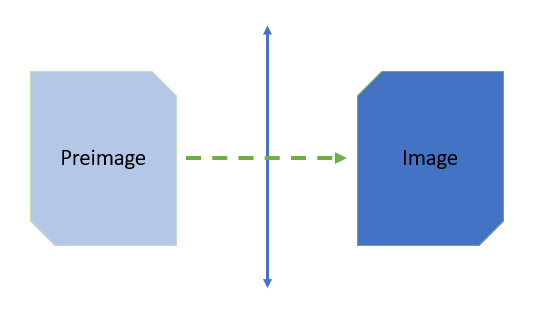# Preimage & Image

Contents:

## Preimage & Image in GeometryThe shape on the left (the preimage) is reflected over the y-axis to the shape on the right (the image).

In geometry, figures in a plane can be transformed in a variety of ways, including shifts and scaling, to produce new shapes. The new (transformed) shapes are called images and the original, unaltered shapes are called preimages. In other words, a transformation can be though of as a procedure that maps a preimage onto an image.

## Functions Preimage & Image

The idea of an image and a preimage when you’re working with functions is the same idea as translation a shape, only here you’re “translating” sets of numbers. If f(a) = b, then :

• b is the image of a under f (when the function is clear from the context, the “under f” part is often dropped).
• a is an element of the preimage of b.

Images are elements of the range and preimages are subsets of the domain. Therefore, there may be more than one preimage of b, but only one image of a. This is just an extension of what you probably already know about functions: they must be “one to one” or “many to one” but cannot be “many to one”.

## Properties of Images and Pre-Images

In the following list of properties, f: A → B indicates than f is function (or map) from A to B:

1. If f : A → B then
• f [A] = ∅
• f -1[B] = ∅.
2. If f : A → B and X, Y ⊆ B then f-1[X ∩ Y] = f-1[X] ∩ f-1[Y].
3. If f: A → B and X, Y ⊆ B then f-1[X ∪ Y] = f-1[X] ∪ f-1[Y]
4. If f: A → B and X, Y, ⊆ A then
• f [A ∪ B] = f|A| ∪ f |B|
• f [A ∩ B] ⊆ f|A| ∩ f |B|

As a simple example, consider the function f(x) = √x:

• The image of 5 is √5.
• The preimage of 5 is 25; for ℕ(the set of natural numbers) it is the set of all perfect squares in ℕ .

## References

 Math 310 Functions Handout.
 Kirby, P. Introduction to Functions. Retrieved November 4, 2021 from: https://www.math.fsu.edu/~pkirby/mad2104/SlideShow/s1_2.pdf

CITE THIS AS:
Stephanie Glen. "Preimage & Image" From StatisticsHowTo.com: Elementary Statistics for the rest of us! https://www.statisticshowto.com/preimage-image/